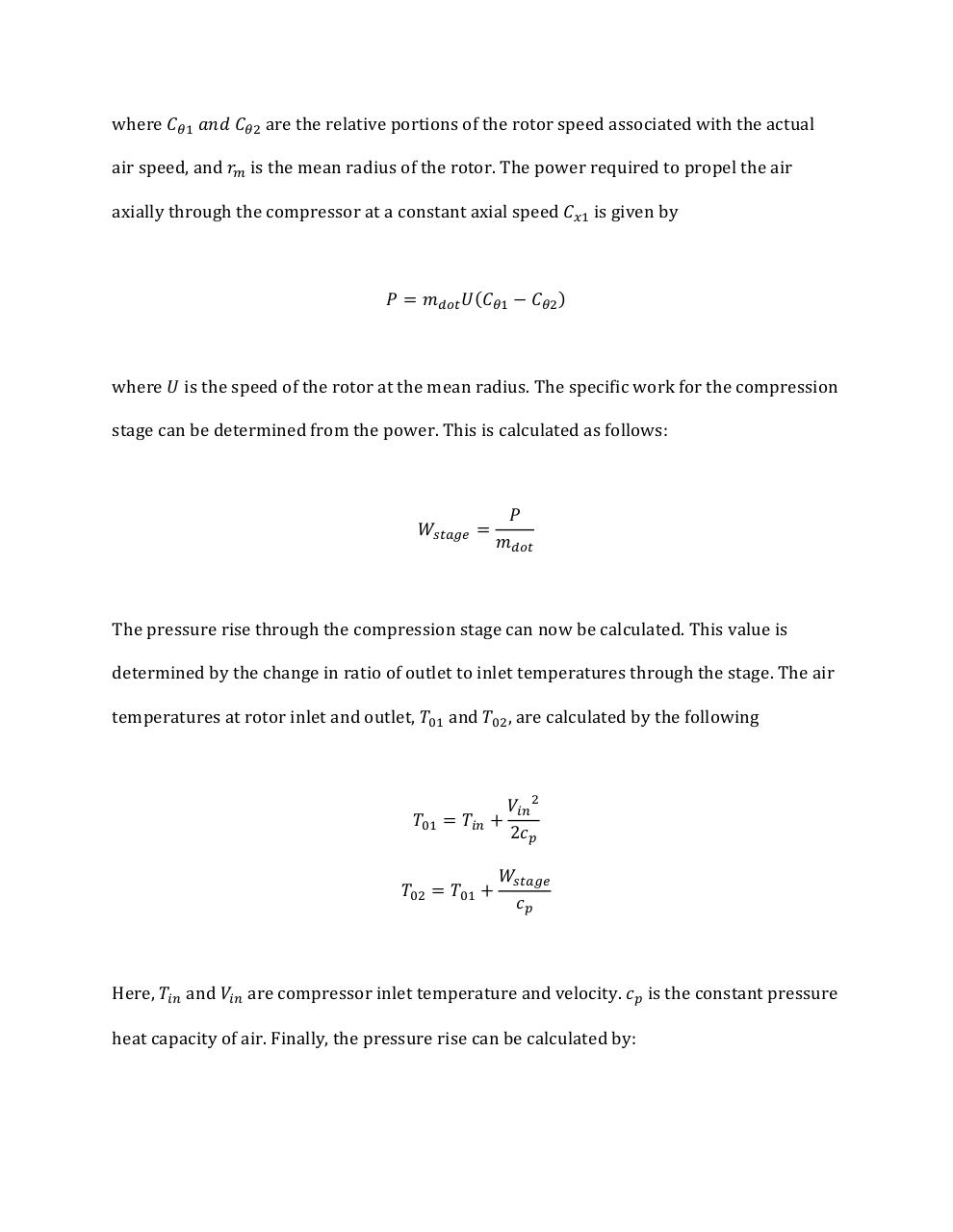# midterm comp.pdfPage 1 2 3 4 5 6

#### Text preview

where 𝐶𝜃1 𝑎𝑛𝑑 𝐶𝜃2 are the relative portions of the rotor speed associated with the actual
air speed, and 𝑟𝑚 is the mean radius of the rotor. The power required to propel the air
axially through the compressor at a constant axial speed 𝐶𝑥1 is given by

𝑃 = 𝑚𝑑𝑜𝑡 𝑈(𝐶𝜃1 − 𝐶𝜃2 )

where 𝑈 is the speed of the rotor at the mean radius. The specific work for the compression
stage can be determined from the power. This is calculated as follows:

𝑊𝑠𝑡𝑎𝑔𝑒 =

𝑃
𝑚𝑑𝑜𝑡

The pressure rise through the compression stage can now be calculated. This value is
determined by the change in ratio of outlet to inlet temperatures through the stage. The air
temperatures at rotor inlet and outlet, 𝑇01 and 𝑇02 , are calculated by the following

𝑇01 = 𝑇𝑖𝑛 +
𝑇02 = 𝑇01 +

𝑉𝑖𝑛 2
2𝑐𝑝

𝑊𝑠𝑡𝑎𝑔𝑒
𝑐𝑝

Here, 𝑇𝑖𝑛 and 𝑉𝑖𝑛 are compressor inlet temperature and velocity. 𝑐𝑝 is the constant pressure
heat capacity of air. Finally, the pressure rise can be calculated by: